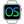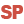UseRulesCalculation rulesNotesDisplaying the resultEquivalence WINDEVWEBDEVWINDEV MobileOthersArithmetic operators Use The arithmetic operators are:"+": Addition (numeric value or string)"-": Subtraction (numeric value)"*": Multiplication"/": Division"++": Increment (numeric value)"--": Decrement (numeric value)"+=": Adding a value to the variable or to the control (numeric or text)"-=": Subtracting a value from the variable or from the control (numeric)Modulo: Returns the remainder of a division"^": Power (equivalent to Power) Rules Calculation rulesThe different calculations are performed without loss of precision and without being truncated. The flow checks are performed when the result is assigned to a variable.Notes Displaying the resultThe result of the calculation can be directly displayed using the following operators:"++": Increment"--": DecrementWhen the ++ (--) operator is used as an expression (e.g.: Info(x++)), its behavior is determined by the position of the operator, relative to the incremented variable:++x (--x) => increments (decrements) x then returns x.x++ (x--) => returns the value of x then increments (decrements) x. For example: let x is int = 5Trace(x++) // Displays 5. x is set to 6 Trace(++x) // Displays 7. x is set to 7Trace(--x) // Displays 6. x is set to 6Trace(x--) // Displays 6. x is set to 5Trace(x) // Displays 5 The result of the calculation cannot be directly displayed by the following operators:"+=": Adding a value to the variable or to the control (numeric or text)"-=": Subtracting a value from the variable or from the control (numeric)Therefore, this example generates an error during the compilation: num is int = 10Trace(num+=1) To display the result, perform the following modifications: num is int = 10num += 1Trace(num) Equivalencej ++ is equivalent to j = j + 1j -- is equivalent to j = j - 1j += 3 is equivalent to j = j + 3j -= 3 is equivalent to j = j - 3We recommend that you use the following syntaxes: "j ++", "j --", "j +=" and "j -=", that are faster than the usual syntaxes. See also Minimum version required Version 9 This page is also available for…